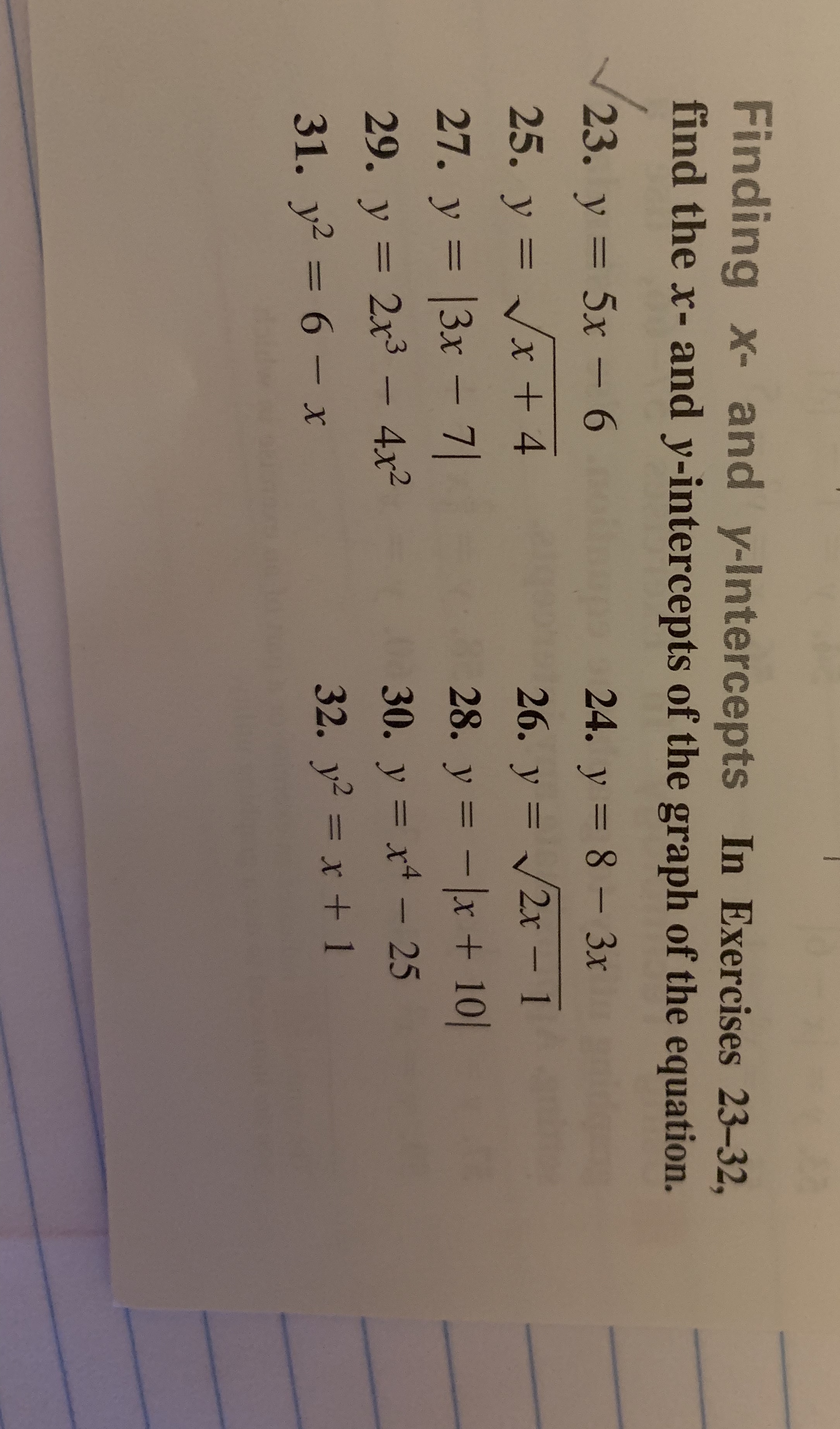# Finding x- and y-Intercepts In Exercises 23-32, find the x- and y-intercepts of the graph of the equation. 23. y = 5x-6 25. y=V/x + 4 24. у=8_3x 28. y =-1x + 101 32. Уг =x + 1 29, y = 2x3-4x2 31. уг-б-х

Question

Find the x and y intercepts of the graph of the equationhelp_outlineImage TranscriptioncloseFinding x- and y-Intercepts In Exercises 23-32, find the x- and y-intercepts of the graph of the equation. 23. y = 5x-6 25. y=V/x + 4 24. у=8_3x 28. y =-1x + 101 32. Уг =x + 1 29, y = 2x3-4x2 31. уг-б-х fullscreen

### Want to see this answer and more?

Experts are waiting 24/7 to provide step-by-step solutions in as fast as 30 minutes!*

*Response times may vary by subject and question complexity. Median response time is 34 minutes for paid subscribers and may be longer for promotional offers.
Tagged in
Math
Algebra

### Applications of Mathematics